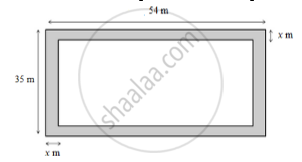# A Footpath of Uniform Width Runs All Around the Inside of a Rectangular Field 54m Long and 35 M Wide. If the Area of the Path Is 420m^2 , Find the Width of the Path. - Mathematics

A footpath of uniform width runs all around the inside of a rectangular field 54m long and 35 m wide. If the area of the path is   420m^2 , find the width of the path.

#### Solution

Area of the rectangular field =54xx35=1890m^2

Let the width of the path be x m. The path is shown in the following diagram:Length of the park excluding the path=(54-2x)m

Breadth of the park excluding the path=(35-2x)m

Thus, we have:

Area of the path=420m^2

⇒420=54xx35-(54-2x)(35-2x)

⇒420=1890-(1890-70x-108x+4x)^2

⇒420=4x^2+178x

⇒4x^2-178x+420=0

⇒2x^2-89x+210=0

⇒2x^2-84x-5x+210=0

⇒2x(x-42)-5(x-42)=0

⇒(x-42)(2x-5)=0

⇒x-42=0  or  2x-5=0

⇒x=42  or  x=2.5

The width of the path cannot be more than the breath of the rectangular field.

∴ x=2.5 m

Thus, the path is 2.5m wide.

Concept: Area of Circle
Is there an error in this question or solution?

#### APPEARS IN

RS Aggarwal Secondary School Class 10 Maths
Chapter 17 Perimeter and Areas of Plane Figures
Q 12